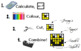# Colouring by Volume and Surface Area, Batman vs Superman (Two 12-Sheet Mosaics)Subject
Resource Type
File Type

Zip

(2 MB|53 pages)
Standards
• Product Description
• StandardsNEW
Volumes of fun will surface with this mathy twist on an epic battle of justice! Two 12-sheet mosaics are included (Superman and Batman), so you can split up your students and have a right-angled colouring showdown! May the best coloured hero win!

This task makes a terrific unit summative assessment. It was designed with the grade 9 academic course (MPM1D Ontario Curriculum) in mind, and includes the volume and surface area of prisms, pyramids, cones, cylinders, and spheres.

CHECK OUT THE PRODUCT PREVIEW to see the range of problem types and know exactly what these worksheets involve!

Each mosaic consists of 12 worksheets (each sheet unique!) involving surface area and volume real-life application word problems. Students' sheets combine to create the hero mosaics. The collaborative motivation is huge! The answers are given in a randomized list at the bottom of each sheet, allowing students to monitor their own work. Complete answer keys are provided for you, as well as a Teaching Tips sheet for smooth implementation!

The Superman vs Batman battle is also available in the following versions:
Fractions - +,-, ×, and ÷ positive mixed fractions
Sine and Cosine Laws - solve for sides and angles
Trig Ratios (SohCahToa) - solve for sides and angles
Linear Systems - substitution, elimination, and graphing
Pythagorean Theorem - Application Word Problems

It's simple!
2. Colour the squares.
4. Combine with the class!

Students complete the problems to decode the colour-by-number key, then colour their section of the mosaic.

INCLUDED:
-.pdf and .docx format of both mosaic files in zip folder
-A Teaching Tips page
-Complete answer keys for quick student assessment of all worksheets
-Answer-range keys (i.e. "Blue always has an answer between 120 and 160")
-Completed colour mosaic guides with coordinates labelled for easy assembly
-A "Problem Order" list, giving the order of problem types followed by all worksheets.

THE MATH INVOLVED:
-Interpret V and SA word problems arising from real life contexts
-Use volume formulas for cylinders, prisms, pyramids, cones, and spheres to solve problems
-Work with fractional and decimal edge lengths in the context of solving real-world problems
-Use both metric and imperial units of measurement for length, area, and volume, and consider the appropriateness of the (randomly chosen) unit for each problem.
-Consider the context of the problem (i.e. does the top area get included?)
-Use the Pythagorean theorem to solve for necessary dimensions (slant height, perpendicular height, apex height)
-Determine desired dimensions given information about the shape (i.e. get radius from circumference, or perpendicular height from a known side length for an equilateral triangle.)

Leave the hero pictures a secret, or let them know their team in advance for motivation… it’s your call.

Encourage students to check their answers by finding them in the randomized list on their worksheet before they colour each square. This will increase the accuracy of the final picture!

All my “Colouring by…” worksheets use standard pencil-crayon colours found in the Crayola 24 pack. For best results, use the exact colour name match (and encourage quality colouring!). Perhaps a class set of pencil crayons would be a fun math department investment!

Feedback, suggestions, and frontline stories are always welcomed!

Thanks for checking this out!
~CalfordMath

If you like this product, be sure the check out the whole Collaborative Math Mosaic directory, sorted by topic!
Use volume formulas for cylinders, pyramids, cones, and spheres to solve problems.
Know the formulas for the volumes of cones, cylinders, and spheres and use them to solve real-world and mathematical problems.
Apply the Pythagorean Theorem to determine unknown side lengths in right triangles in real-world and mathematical problems in two and three dimensions.
Understand that a two-dimensional figure is similar to another if the second can be obtained from the first by a sequence of rotations, reflections, translations, and dilations; given two similar two-dimensional figures, describe a sequence that exhibits the similarity between them.
Solve real-world and mathematical problems involving area, volume and surface area of two- and three-dimensional objects composed of triangles, quadrilaterals, polygons, cubes, and right prisms.
Total Pages
53 pages
Included
Teaching Duration
3 days
Report this Resource to TpT
Reported resources will be reviewed by our team. Report this resource to let us know if this resource violates TpT’s content guidelines.